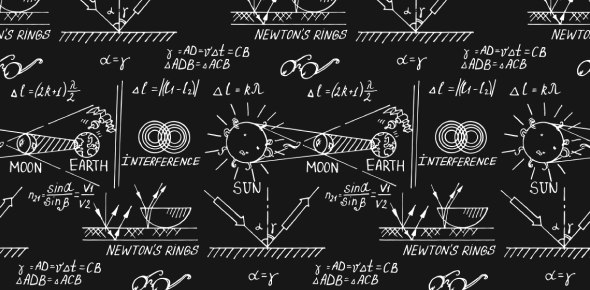# Ch 8 Physics Practice Quiz

12 Questions | Attempts: 473
ShareSettingsRotational speed is calculated by the number of times that an object rotates over the time it rotates. How much do you understand chapter 8 where we got to understand how to calculate this type of speed. Do you believe you understood the introductory part? Take up the physics practice quiz below and test your understanding before the final exam is upon us. All the best and keep practicing what you learn.

• 1.
Define rotational inertia.
• A.

The property of an object to resist change.

• B.

The property of an object to resist change in a linear motion.

• C.

The property of an object to resist change in a rotational motion.

• 2.
Define tangential speed.
• A.

A measurement that measures distance travelled per unit of time in circular motion.

• B.

A measurement that measures the number of rotation per unit of time in a circle motion.

• C.

A MEASUREMENT THAT MEASURES HOW MUCH FORCE WAS APPLIED TO A ROTATION.

• 3.
Circle the correct statement based on this situation. You apply 27N of force on a wrench.
• A.

As you decrease the radius where you apply the force, you will increase the torque of the wrench/

• B.

As you increase the radius where you apply the force, you will decrease the torque of the wrench.

• C.

As you decrease the radius where you apply the force, you will decrease the torque of the wrench.

• 4.
Put a pipe over the end of a wrench when trying to turn a stubborn nut on a bolt, to effectively make the wrench handle twice as long, you'll multiply the torque by...
• A.

1

• B.

2

• C.

4

• 5.
On a merry-go-round, horse A has a radius from the center of 10m while horse B has a radius from the center of 15m. Which horse has a greater rotational speed?
• A.

Horse A

• B.

Horse B

• C.

Neither

• 6.
Toss a baseball bat into the air and it wobbles about its
• A.

Geometrical center

• B.

Center of mass

• C.

Heavier end

• 7.
When a twirling ice skater brings her arms inward, her rotational speed
• A.

Increases

• B.

Decreases

• C.

Remains the same

• 8.
As rotational speed of a merry-go-round increases by 2, what will happen to tangential speed?
• A.

Decrease by 1/4

• B.

Decrease by 1/2

• C.

Increase by 2

• 9.
At point C on the merry-go-round the tangential speed is .2 m/s how can point C double the speed to .4m/s
• A.

Increase rotational speed by 2

• B.

Decrease the rotational speed by 1/2

• C.

Increase the rotational speed by 4

• 10.
A torque acting on an object tends to produce
• A.

Equillibrium

• B.

Rotation

• C.

Linear motion

• 11.
On a balanced see-saw, a cow is three times as heavy as his partner sits
• A.

1/3 the distance from the fulcrum

• B.

Less than 1/3 the distance from the fulcru.

• C.

More than 1/3 the distance from the fulcrum

• 12.
Two people are balanced on a see-saw. If one person leans toward the center of the see-saw, that person's end of the see-saw will
• A.

Rise

• B.

Fall

• C.

Stay at the same level

## Related TopicsBack to top
×

Wait!
Here's an interesting quiz for you.